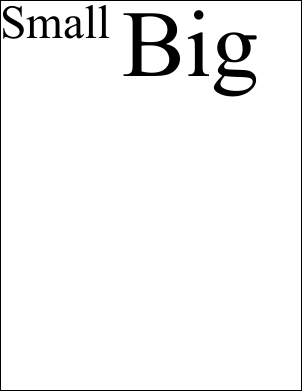Type Default Read Only Description [C#] int [Visual Basic] Integer 10 No The current text size.Notes

 The line height for drawing text. This property determines the size of text that is added to the document using methods like AddText. The font size is measured in the current Units. You should prefer use of the XTextStyle.Size property, to which the FontSize property is simply an integer accessor. Assigning a value to the FontSize is exactly equivalent to assigning it to the XTextStyle.Size. Getting a value from this property is exactly equivalent to the following.   int n = (int)(doc.TextStyle.Size + 0.5); Dim n As Integer = CInt((doc.TextStyle.Size + 0.5))Example

 The following example adds two blocks of styled text to a document. The first block is in 96 point type and the second is in 192 point type.   using (Doc doc = new Doc()) {   doc.FontSize = 96;   doc.AddText("Small ");   doc.FontSize = 192;   doc.AddText("Big");   doc.Save(Server.MapPath("docfontsize.pdf")); } Using doc As New Doc()   doc.FontSize = 96   doc.AddText("Small ")   doc.FontSize = 192   doc.AddText("Big")   doc.Save(Server.MapPath("docfontsize.pdf")) End Usingdocfontsize.pdf